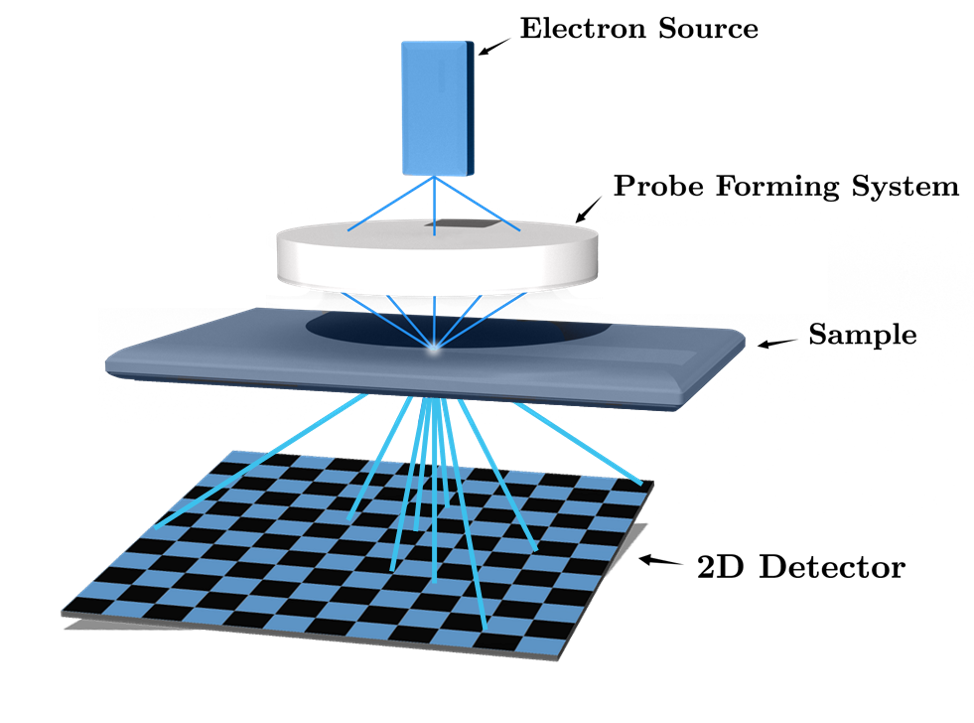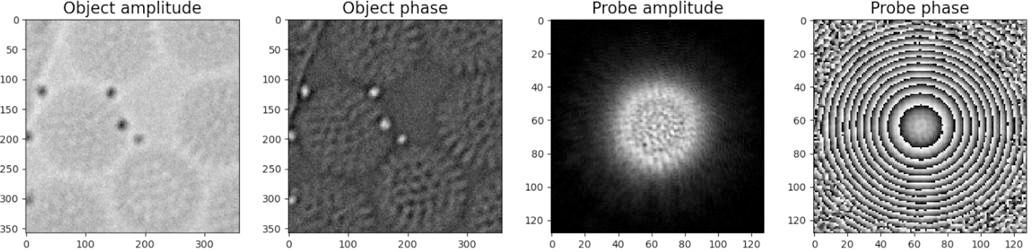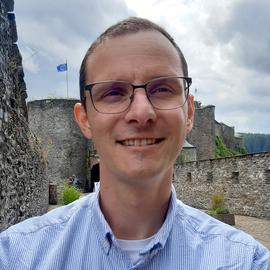# Phase retrieval for 4-D Scanning Transmission Electron Microscopy (4D - STEM)

Many applications observe a signal or an image in an indirect way, through its frequency-or Fourier-representation. For instance, this means that rather than observing individual pixels of an image, we acquire the spectral content of this image, its Fourier transform. This situation occurs, for example, in magnetic resonance imaging (MRI), interferometric radio-astronomy, Fourier transform imaging, Electron Ptychography, and 4-D scanning transmission electron microscopy (or STEM).Figure 1: Working principle of 4-D STEM.Figure 2: Reconstruction of both object and probe from 4-D STEM data of a rotavirus (data credits ).

However, while a full description of this spectral content requires a complex-valued representation of the acquired frequencies (with amplitudes and phases), most sensors can only measure intensities (squared amplitude); the phase information is thus lost and recovering the observed signal or image becomes challenging.

Mathematically, in the case of a $$n$$ -sample signal $$x\in \mathbb R^{n} \times \mathbb{R}^{n}$$ (or a 2-D image with $$n$$ pixels), this means that we observe the amplitude of its Fourier transform

\begin{equation} |\mathcal F{x}(\omega)|^{2} = |\langle x,z_i\rangle |^{2} \end{equation}

with the vector $$z_{i} \in \mathbb C^{n}$$ representing the $$i$$-th element of the Fourier basis $$\mathcal F$$, that is the vector $$z_{i}$$ with components $$z_{ik} = \exp⁡(2\pi j ik/n)/ \sqrt{n}$$ and (discrete) frequency $$\omega = 2\pi i / n$$

Recovering the signal $$x$$ from all $$m$$ phase-less measurements $$\{|\langle x, z_{i} \rangle|^{2}\}_{i=1}^{m}$$ is a challenging ill-posed, non-linear inverse problem. Under certain conditions, it can be solved either using specific non-convex optimizations schemes, for instance by running a gradient descent over the minimization

\begin{equation} \min_{u} \sum_{i} \Big| |\langle x, z_{i} \rangle|^{2} - |\langle u, z_{i} \rangle|^{2} \Big|^{2}\ \text{such that}\ u \in \mathcal{S} \tag{1} \end{equation}

where $$\mathcal S$$ represents some structural information we know a priori about $$x$$ (such as its sparsity )

Four-dimensional Scanning Transmission Electron Microscopy (4-D STEM) amounts to a variant of the phase retrieval problem. In particular, 4-D STEM aims at imaging the atomic structure of organic and non-organic samples (see the figure above). It relies on recording two-dimensional (2-D) diffraction patterns (that is, a Fourier representation), in intensity, for every position of a convergent electron beam . In combination with algorithms that solve the phase retrieval problem, a 4-D STEM dataset allows the reconstruction of a high-resolution object, by exploiting the high amount of redundancy in the data.

A simplified acquisition model for 4-D STEM is as follows. Given a (complex) object $$x$$ we want to image (that is, the object atomic structure, see above), a window or probe $$w_{p}$$ representing the electron beam intensity localized at 2-D position $$p$$ on the sample, we collect the four-dimensional object:

\begin{equation} y(\omega,p) = |\mathcal F\{x\cdot w_p\}(\omega)|^{2}, \tag{2} \end{equation}

where $$\omega$$ is the 2-D frequency measured in the 2-D detector, and $$\mathcal F$$ is now the 2-D Fourier transform inducing the diffraction pattern.

This sensing model is thus a variation of the phase retrieval question where one probes the object $$x$$ only over a localized window (as induced by the probe $$w_{p}$$ representative of the focused electron beam).

What makes 4-D STEM challenging is the fact that both object $$x$$ and the probe $$w_p$$ are unknown (this probe is, however, translation invariant; its shape stays the same, it’s only translated on $$p$$) [3,4].

Master project objectives: The objective of this master project is to design algorithms to recover both $$x$$ and the probe $$p$$ from the phase-less observations provided by the model (2). The master project is structured according to the following steps with increasing levels of difficulty (the objective is not, however, to complete all of these; it rather provides a research direction):

1. Developing an algorithm for the recovery (from Eq. (1)) of 1-D signal from phase-less Fourier observations when the probe is known and when all the required data are collected;
2. Extending this algorithm to the blind case when the probe is unknown or when the 4-D data is partially available;
3. Generalizing the problem to 2-D images in a synthetic context;
4. Applying this method to actual STEM measurements.

During the project, the interested student will have to:

• learn fundamental aspects of inverse problem solving via non-convex optimization (using gradient descent or more advanced first order optimization methods),
• understand how one can ease the inverse problem solving by assuming simple models over the object of interest (such as sparsity or low-rankness); this is called inverse problem regularization;
• efficiently programming non-convex optimization in Python using known toolboxes (PyCVX, SciPy, PyWavelets, …)
• objectively quantifying the quality of the estimated object/probe and the efficiency of the proposed algorithms.

This master project will be co-supervised by Prof. Laurent Jacques (UCLouvain) and Dr. Amirafshar Moshtaghpour (Rosalind Franklin Institute & Mechanical, Materials, & Aerospace Engineering, University of Liverpool, UK).

References:

•  J. Rodenburg and A. Maiden in “Springer handbook of microscopy”, eds. PW Hawkes and JCH Spence (Springer International Publishing, Cham, Switzerland), pp. 819-904.
•  Y. C. Eldar, P. Sidorenko, D. G. Mixon, S. Barel, O. Cohen, “Sparse Phase Retrieval from Short-Time Fourier Measurements”, https://arxiv.org/abs/1411.1380
•  Four-dimensional Scanning Transmission Electron Microscopy, https://www.youtube.com/watch?v=OhgFlhbURzs
•  Zhou, L., Song, J., Kim, J. S., Pei, X., Huang, C., Boyce, M., & Wang, P. (2020). Low-dose phase retrieval of biological specimens using cryo-electron ptychography. Nature communications, 11(1), 1-9.
•  Jacques, L., Duval, L., Chaux, C., & Peyré, G. (2011). A panorama on multiscale geometric representations, intertwining spatial, directional and frequency selectivity. Signal Processing, 91(12), 2699-2730. https://arxiv.org/abs/1101.5320